Examples

Chapter 15 Class 10 Probability
Serial order wise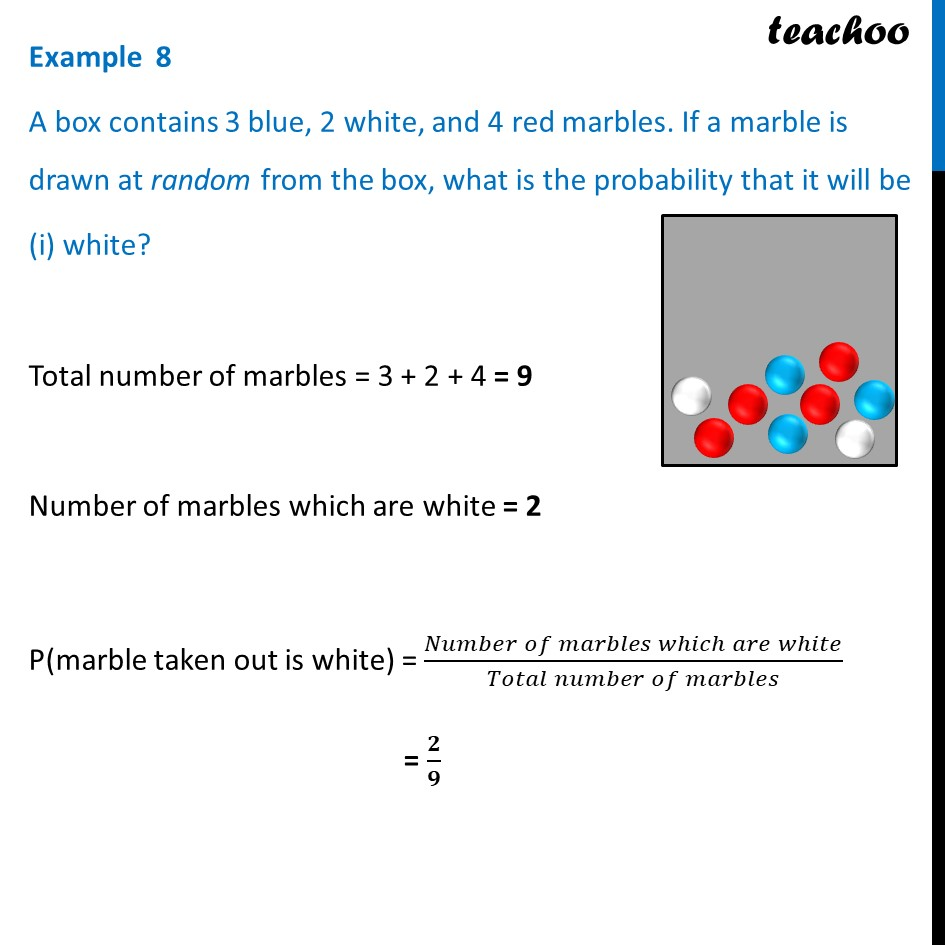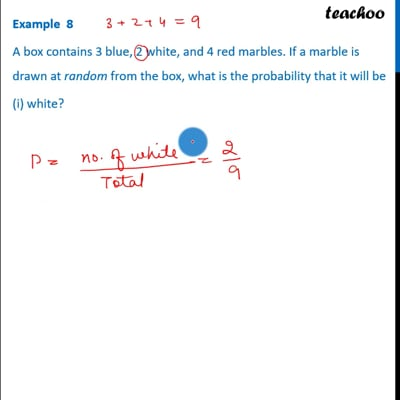This video is only available for Teachoo black users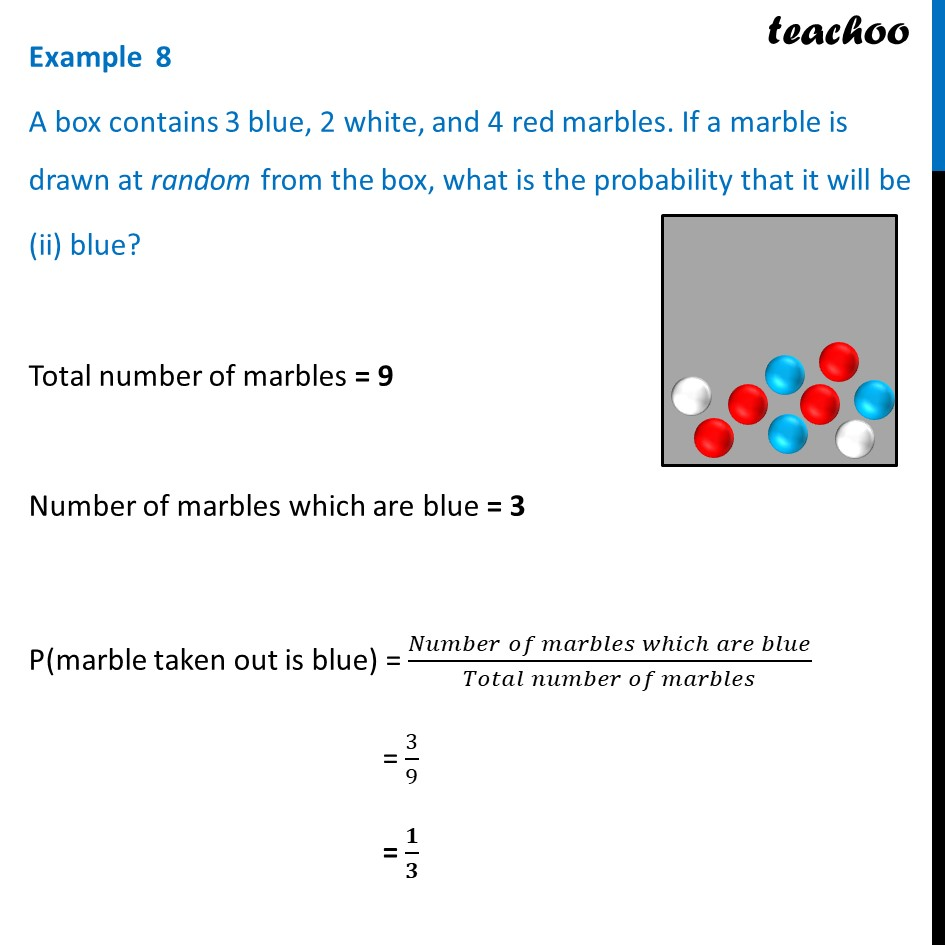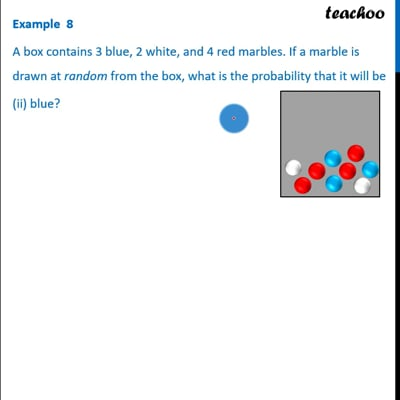This video is only available for Teachoo black users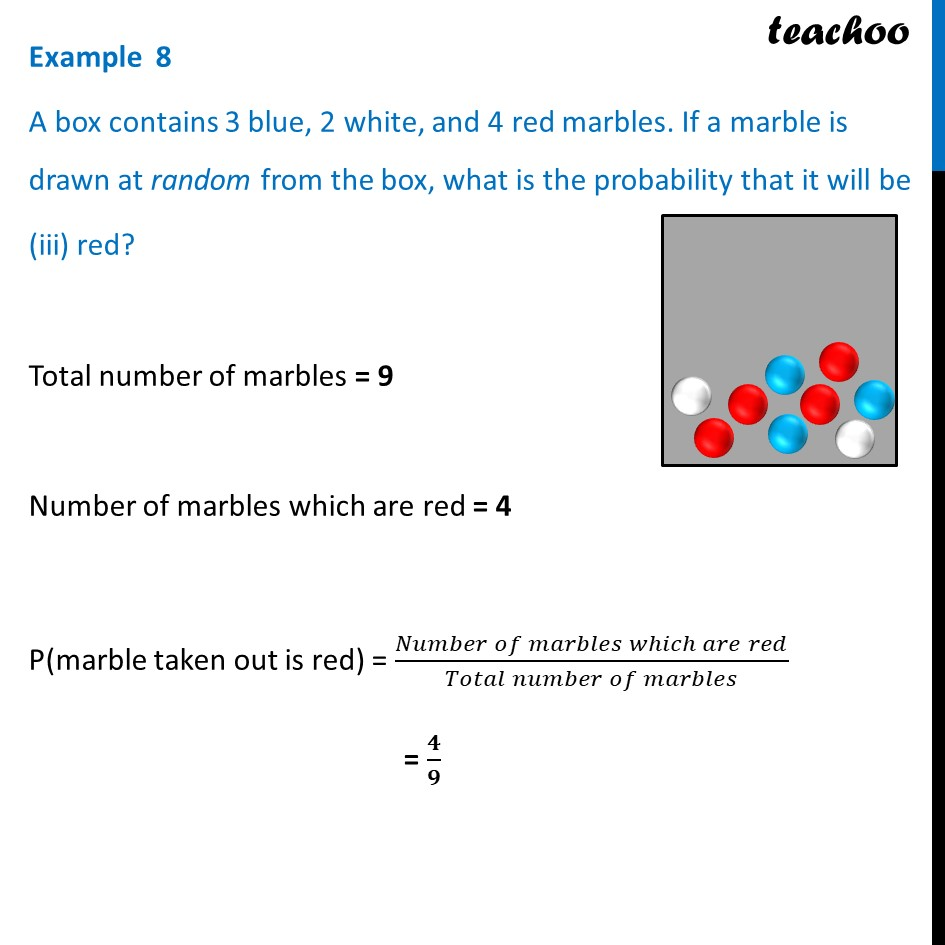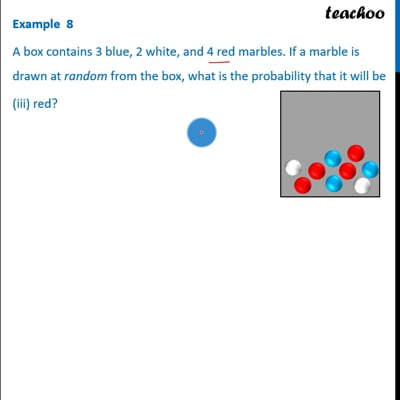This video is only available for Teachoo black users

Introducing your new favourite teacher - Teachoo Black, at only ₹83 per month

### Transcript

Example 8 A box contains 3 blue, 2 white, and 4 red marbles. If a marble is drawn at random from the box, what is the probability that it will be (i) white? Total number of marbles = 3 + 2 + 4 = 9 Number of marbles which are white = 2 P(marble taken out is white) = (𝑁𝑢𝑚𝑏𝑒𝑟 𝑜𝑓 𝑚𝑎𝑟𝑏𝑙𝑒𝑠 𝑤ℎ𝑖𝑐ℎ 𝑎𝑟𝑒 𝑤ℎ𝑖𝑡𝑒)/(𝑇𝑜𝑡𝑎𝑙 𝑛𝑢𝑚𝑏𝑒𝑟 𝑜𝑓 𝑚𝑎𝑟𝑏𝑙𝑒𝑠) = 𝟐/𝟗 Example 8 A box contains 3 blue, 2 white, and 4 red marbles. If a marble is drawn at random from the box, what is the probability that it will be (ii) blue? Total number of marbles = 9 Number of marbles which are blue = 3 P(marble taken out is blue) = (𝑁𝑢𝑚𝑏𝑒𝑟 𝑜𝑓 𝑚𝑎𝑟𝑏𝑙𝑒𝑠 𝑤ℎ𝑖𝑐ℎ 𝑎𝑟𝑒 𝑏𝑙𝑢𝑒)/(𝑇𝑜𝑡𝑎𝑙 𝑛𝑢𝑚𝑏𝑒𝑟 𝑜𝑓 𝑚𝑎𝑟𝑏𝑙𝑒𝑠) = 3/9 = 𝟏/𝟑 Example 8 A box contains 3 blue, 2 white, and 4 red marbles. If a marble is drawn at random from the box, what is the probability that it will be (iii) red? Total number of marbles = 9 Number of marbles which are red = 4 P(marble taken out is red) = (𝑁𝑢𝑚𝑏𝑒𝑟 𝑜𝑓 𝑚𝑎𝑟𝑏𝑙𝑒𝑠 𝑤ℎ𝑖𝑐ℎ 𝑎𝑟𝑒 𝑟𝑒𝑑)/(𝑇𝑜𝑡𝑎𝑙 𝑛𝑢𝑚𝑏𝑒𝑟 𝑜𝑓 𝑚𝑎𝑟𝑏𝑙𝑒𝑠) = 𝟒/𝟗